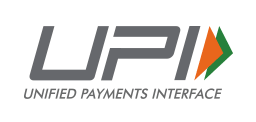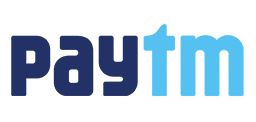Sale!

# MCS-011 Problem Solving and Programming Solved Assignment 2022-23

30.00

 Course Code MCS-011 Course Title Problem Solving and Programming Assignment Code BCA(2)/011/Assignment/2022-23 Session 2022-23 Submission Date 31st October, 2022 (For July Session) 15th April, 2023 (For Jan Session)

Features

• Complete Assignments
• Lowest Price
• Secure Payments
GUARANTEED SAFE CHECKOUT
••## Description

MCS-011 Solved assignment 2022-23 | IGNOU BCA 2nd Semester Solved Assignment 2022-23 | www.techiya.in

Q1. Write an algorithm, draw corresponding flow chart and write an interactive program in C which prompts the user with the following options on the opening menu:
1) To reverse a 5-digit number
2) To check whether the 5-digit number is a palindrome or not
3) To find the sum of all the digits of the 5-digit number
4) Quit
Note: Use SWITCH..CASE statement.

Q2. Write the functions in C for the following:
(a) To calculate the power without using the pow(x,y) function of <;math.h>.
(b) To calculate the cube of a given number.
(c) To calculate the LCM of two given numbers

Q3. Write interactive programs in C using recursion for the following:
(a) To find the HCF of two given numbers.
(b) To find the GCD of two given numbers.
(c) To find the factorial of a given number.

Q4. Write interactive C programs to perform the following on strings:
(a) To check if two strings are Anagram.
(b) To check whether the given alphabet is a vowel or consonant.
(c) To convert the given string from lower case to upper case without using the inbuilt function in <string.h>

Q5. Using arrays in C write a program to input 2 arrays consisting of 10 numbers each and display all the numbers those are common to both the arrays and display their count.

Q6. Using Structures in C, write an interactive program to display the pay-slips for 10 employees working in a small departmental stores.
Note: Assumptions can be made wherever necessary and mention them.

Q7. Using File Handling concept in C programming, write the C programs for the following:
(a) To create and store student information of 10 MCA students in a .dat file.
(b) To edit the file created in (a) to add data for 2 more students.
(c) To delete specific line(one student record) from a file.

## Reviews

There are no reviews yet.